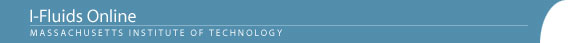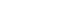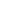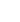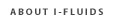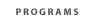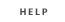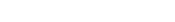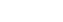2.25: Advanced Fluid Dynamics Section 1: Continuum viewpoint and the equation of motion Section 2: Static Fluids Section 3: Mass Conservation Section 4: Inviscid flow - differential approach Section 5: Control Volume Theorums Section 6: Navier-Stokes equation and viscous flow Section 7: Similarity and dimensional analysis Section 8: Boundary layers, separation and effect on drag/lift Section 9: Vorticity and circulation Section 10: Potential flows; lift, drag and thrust production Section 11: Surface tension and its effect on flows Section 12: Introduction to turbulence Back to 2.25 HomeSection 4: Inviscid Flow I: Euler's Equation of Motion, Bernoulli's Integral, and the Effects of Streamline Curvature4.1 Euler's equation for inviscid motion: a relationship between fluid acceleration (convective and temporal) and pressure distribution.
4.2 Concepts for describing fluid flows: streamlines, particle paths, and streaklines.
4.3 Euler's equation for steady flow expressed in streamline coordinates: the pressure-velocity relation along the streamline direction, and the pressure gradient normal to streamlines when streamlines have curvature. Comments on the "inviscid" flow approximation and the boundary conditions that are appropriate for velocity and pressure in such flows.
4.4 Incompressible flow examples involving both Bernoulli's integral and the effects of streamline curvature.  Classroom demonstrations of the Bernoulli effect and the streamline curvature effect (the latter being the origin of lift on airfoils).
4.5 Bernoulli's integral for two types of steady, isentropic, compressible flows:  (a) perfect gases and (b) liquids with constant compressibility.  Isentropic expansion of a gas into a vacuum.  A criterion for "incompressible flow.
4.6 The general form of Bernoulli's integral for unsteady flow.  Examples: startup problems, Rayleigh bubble oscillations, etc., mainly for incompressible flows.
4.7 Introductory comments on potential (vorticity-free) flow and the velocity potential f.  Incompressible flows as solutions of Ñ2f = 0 with f/n =0 at solid boundaries.  The equation for pressure in terms of the velocity potential f

Reading
Fay, Chapter 4

Problem Set Section 4

Problem 4.1
Problem 4.4
Problem 4.8
Problem 4.9
Problem 4.15
Problem 4.18
Problem 4.19
Problem 4.21
Problem 4.23
Problem 4.24
Problem 4.28